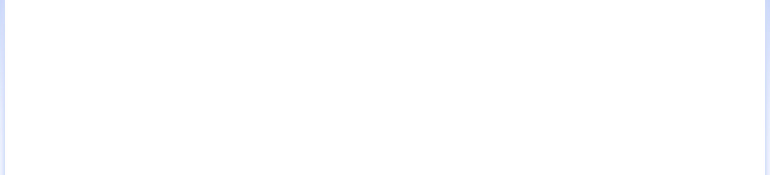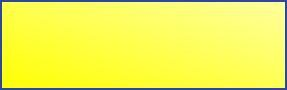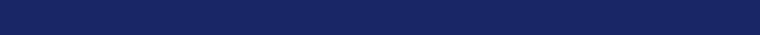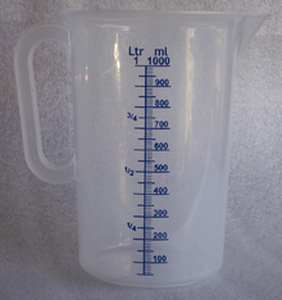MedFriendly®Milliliter
A milliliter (sometimes written as millilitre) is a
measurement of volume that is equal to one
thousandth of a liter, meaning that it takes one
thousand milliliters to equal one liter. A liter is a
measurement of the amount of space that a liquid
takes up in a container, which is equal to
1.056688 quarts.

To understand this better, picture a gallon of milk.
It takes 4 quarts of milk to make up one gallon of
milk. Since one liter is a little bit more than one
quart, 4 liters of milk is a little bit more than one
gallon of milk.

FEATURED BOOK: Oxford Dictionary of Weights, Measures, and Units

Now that you know how much is in a liter, you can tell that a milliliter is a very small
amount of liquid. The measurements stated above are based on those used in the United
States. Milliliters are often used in healthcare to measure amounts on liquid medicine.
There are about 5 (4.92) milliliters in one teaspoon and 29.57 milliliters to make one fluid
ounce. Milliliter is often abbreviated as mL or as 1cm3. Milliliter comes from the Greek
word "milli" meaning "one thousand," and the Greek word "litra" meaning "pound." Put the
two words together and you have "one thousand(th) (of a) pound."
"Where Medical Information is Easy to Understand"™